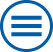## The group contributes to the following mathematical research topics of WIAS:

Direct and inverse problems for the Maxwell equations

The work is focussed on models for inductive heating of steel and for light scattering by periodic surface structures. For this the quasi-stationary Maxwell equation is coupled with nonlinear partial differential equations and the timeharmonic Maxwell equation is combined with special radiation conditions, respectively. The convergence of numerical methods and several inverse promblems are analyzed. [>> more]

Direct and inverse problems in thermomechanics

Thermomechanical models are the basis for the description of numerous technological processes. The consideration of phase transitions and of inelastic constitutive laws raise exciting new questions regarding the analysis of direct problems as well as the identification of materials parameters. [>> more]

Numerical Methods for PDEs with Stochastic Data

Models of real-world phenomena inevitably include uncertainties which influence the solutions in a nonlinear way. Numerical methods for PDE with stochastic data enable to quantify such uncertainties of the solution in dependence of the stochastic inputdata. [>> more]

Optimal control of partial differential equations and nonlinear optimization

Many processes in nature and technics can only be prescribed by partial differential equations,e.g. heating- or cooling processes, the propagation of acoustic or electromagnetic waves, fluid mechanics. Additionally to challenges in modeling, in various applications the manipulation or controlling of the modeled system is also of interest in order to obtain a certain purpose... [>> more]

Statistical inverse problems

In many applications the quantities of interest can be observed only indirectly, or they must be derived from other measurements. Often the measurements are noisy and the reconstruction of the quantities of interest from noisy measurements is unstable. [>> more]

Stochastic Optimization

Stochastic Optimization in the widest sense is concerned with optimization problems influenced by random parameters in the objective or constraints. [>> more]